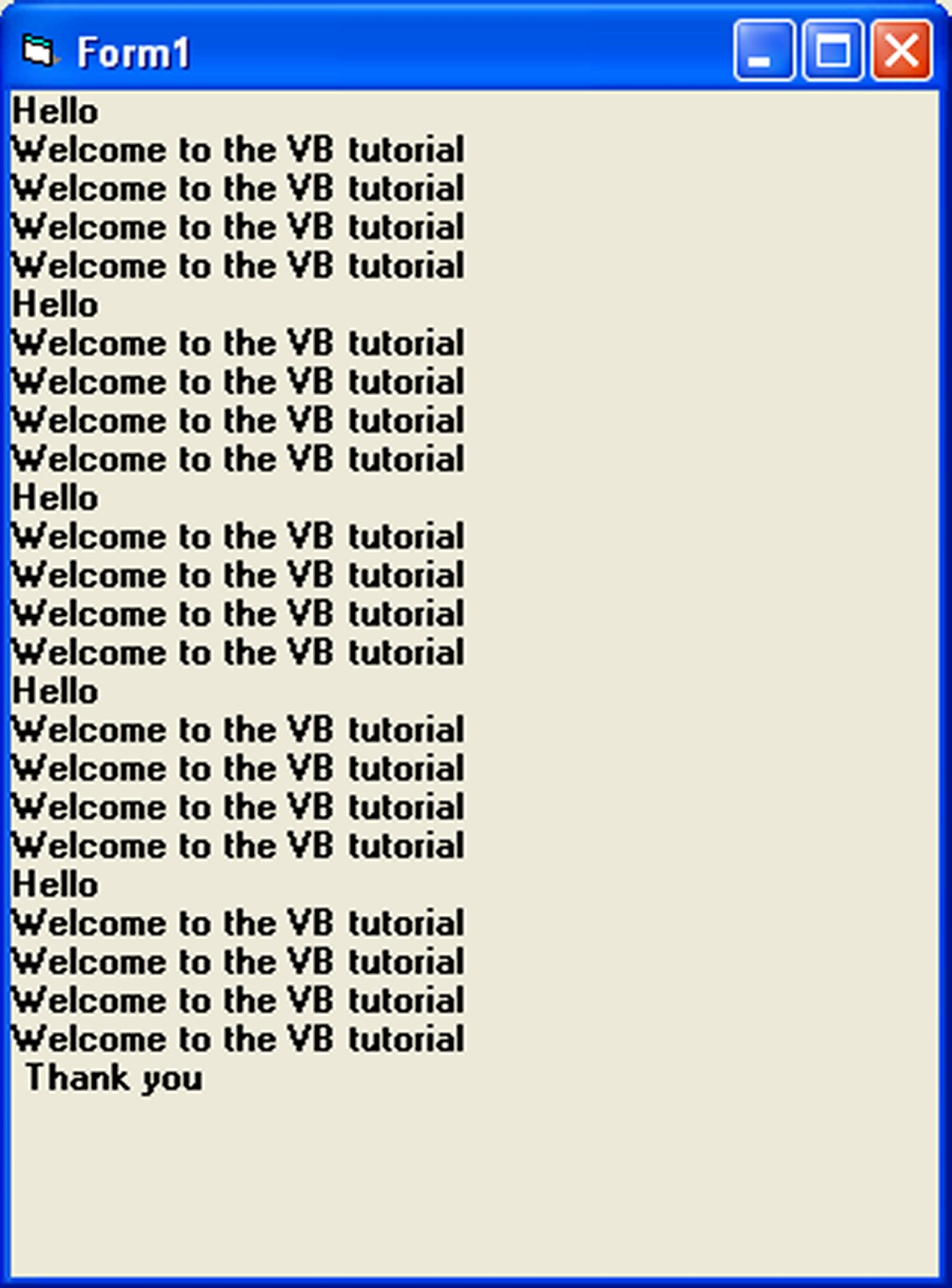# Lesson 9 : Looping

We can create a Visual Basic procedure that enables the program to run iteratively until specific conditions are satisfied. This procedure is commonly referred to as looping. Looping is an invaluable feature of Visual Basic as it simplifies repetitive tasks and enhances efficiency. There are  three kinds of loops in Visual Basic,  the Do...Loop ,the For.......Next loop and the While.....Wend Loop.

### 9.1 The Do Loop

The Do Loop statements have four different forms, as shown below:

##### a)
``` Do While condition
Block of one or more VB statements
Loop```
##### b)
``` Do
Block of one or more VB statements
Loop While condition ```
##### c)
``` Do Until condition
Block of one or more VB statements
Loop ```
##### d)
```Do
Block of one or more VB statements
Loop Until condition
```

#### Example 9.1

```Do while
counter <=1000
num.Text=counter
counter =counter+1
Loop
```

* The above example will keep on adding until counter > 1000

The above example can be rewritten as

```Do
counter=counter+1
Loop until counter>1000
```

### 9.2 Exiting the Loop

Sometime we need exit to exit a loop earlier when a certain condition is fulfilled. The keyword to use is  Exit Do. You can examine Example 9.2 for its usage.

#### Example 9.2

```Dim sum, n as Integer
Private Sub Form_Activate()
List1.AddItem "n" & vbTab & "sum"
Do
n=n+1
sum=sum+n-resize
List1.AddItem n & vbTab & sum
If n=100 Then
Exit Do
End If
Loop
End Sub
```

Explanation

In the above example, we compute the summation of 1+2+3+4+……+100.  In the design stage, you need to insert a ListBox into the form for displaying the output, named List1. The program uses the AddItem method to populate the ListBox. The statement List1.AddItem "n" & vbTab & "sum" will display the headings in the ListBox, where it uses the vbTab function to create a space between the headings n and sum.

#### 9.3 The For....Next Loop

The For....Next Loop event procedure is written as follows:

```For counter=startNumber to endNumber (Step increment)

One or more VB statements

Next ```

#### Example 9.3 a

```
Private Sub Command1_Click()
Dim counter As Integer
For counter = 1 To 10
Next
End Sub
```

#### Example 9.3 b

```Private Sub Command1_Click()

Dim counter As Integer
For counter = 1 To 1000 Step 10
counter = counter + 1
Print counter
Next
End Sub

```

#### Example 9.3 c

```For counter=1000 to 5 step -5
counter=counter-10
If counter<50 then
Exit For
Else
Print "Keep Counting"
End If
Next```

#### Example 9.3 d

```Private Sub Form_Activate( )
For n=1 to 10
If n>6 then
Exit For
Else
Print n
Enf If
Next
End Sub```

Sometimes the user might want to get out from the loop before the whole repetitive process is executed, the command to use is Exit For. To exit a For….Next Loop, you can place the Exit For statement within the loop; and it is normally used together with the If…..Then… statement. Its usages is shown in Example 9.3 d.

### 9.4 Nested For...Next  Loop

When you have a loop within a loop, then you have created a nested loop. You can actually have as many loops as you want in a nested loop provided the loops are not the never-ending type. For a nested loop that consists of two loops, the first cycle of the outer loop will be processed first, then it will process the whole repetitive process of the inner loop, then the second cycle of the outer loop will be processed and again the whole repetitive process of the inner loop will be processed. The program will end when the whole cycle of the outer loop is processed.

The Structure of a nested loop is :

``` For counter1=startNumber to endNumber (Step increment)
For counter2=startNumber to endNumber (Step increment)
One or more VB statements
Next counter2
Next counter1
```

#### Example 9.4

```Private Sub Form_Activate ( )
For firstCounter= 1to 5
Print "Hello"
For secondCounter=1 to 4
Print "Welcome to the VB tutorial"
Next secondCounter
Next firstCounter
Print"Thank you"
End Sub
```#### Figure 9.1

The output of the above program is shown in Figure 9.1. As the outer loop has five repetitions, it will print the word “Hello” five times. Each time after it prints the word “Hello”, it will print four lines of the “Welcome to the VB tutorial” sentences as the inner loop has four repetitions.

### 9.5 The While….Wend Loop

The structure of a While….Wend Loop is very similar to the Do Loop. it takes the following form:

``` While condition
Statements
Wend
```

The above loop means that while the condition is not met, the loop will go on. The loop will end when the condition is met. Let’s examine the program listed in example 9.4.

#### Example 9.5

```Dim sum, n  As Integer
Private Sub Form_Activate()
List1.AddItem "n" & vbTab & "sum"
While n <> 100
n = n + 1
Sum = Sum + n
List1.AddItem n & vbTab & Sum
Wend
End Sub```